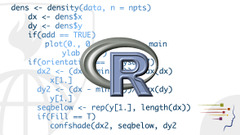## 心得:

• R的資料型態很特別，很”資料導向”． 詳細可以參考這篇R資料格式教學
• R對於資料的操作也很特別，尤其是對於data frame的操作思維是其他語言不容易見到的． 透過 row 跟 col 直接來尋找，取出並且操作．
• 許多函數都相當的直覺，很適合初學者學習．比如說想找有幾筆資料就打nrow()想知道欄位名稱就打colnames()，要算平均就打 mean()．也難怪聽說R都是一些數學很好但是可能是程式設計的初學者．

## 課程筆記:

### 第一週

#### 課程內容:

• vector 產生指令: c( ... )
• 產生出的vector會變成同一個class，不同元素會採取比較寬鬆的class來包括
• 如果你打 x <- c(1, 'a') 則會把全部元素轉成 character (包括 1)
• list 產生指令: list( ... )
• 跟vector不同，每個元素可以是不同class
• 操作方式:
• 給值: x <- list('a', 2, TRUE)
• 取用: x[] # 'a'
• matrix 產生指令: matrix( ... )
• 每一個元素都是integer
• 可以直接給值 x <- matrx(1:6, nrow=2, ncol=3)
• 也可以透過 cbind() (column bind) 或是 rbind() (row bind) 來組合出matrix

x <- list(10, 5, 7, 20, 1, 3)

// 找出 所有 x > 10
x[x > 10]

[]
 20

// 找出 x>10的index
which(x>10)


### 第二週

#### 課程內容:

• Function中可以define function:

    #cube 裡面還有一個 function  n*n
cube<-function(n){
sq<-function() n*n
n*sq()
}

• Free variables

  #指的是並沒有被清楚assign 的變數
cube<-function(n){
sq<-function() n*n
n*sq()+y
}

# 這個例子裡面 y就是 free variables

• Lexical scoping v.s. dynamic scoping

• lexical scoping: free variables resolved to search when function were defined.
• dynamic scoping: free variables resolved to search when function were called.

#### 關於作業

• 作業者要是寫一些function，難度都還算簡單．不過也能充分體會R在處理資料上面的強大支援．
• lapply 的功能很強大，可以把要處理的function 丟進去

  #load_files <- number of file name
# read csv files into tables vector

# get mean value of each table, and also remove NA
lapply(total_tables, mean, na.rm = TRUE)

• NROW(na.omit(one_data_frame)) 可以算出data frame裡面

• 針對data frame 的建立，可以用以下方式丟進for裡面來處理

  #data_length 為某個特定的size
index <- numeric(data_length)
numbers <- numeric(data_length)
for (i in 1: data_length) {
index[i] <- i
numbers[i] <- i + 5 #任意資料
}
ret_data_frame = data.frame(index, numbers, stringsAsFactors=FALSE)

• 這裏有用到計算correlation的函式cor (細節參考)，使用上記得要加上 use = "complete.obs"避免使用到空白欄位．

### 第三週

#### 課程內容

• 對於反覆要對每一個資料表執行的指令，可以透過lapply來執行．
• 底層loop用C，可能會比較快？
• 範例：
• lapply(x_list, mean): 針對x_list的資料，做平均值．（所已lapply回傳也都為一個list)
• apply不一定比for還快，但是打的字可以比較少 (? 講義裡面是這樣講的 XD)
• 範例:
• apply(x, 1, sum) 對x資料中的第一個維度處理”加總”．
• mapply可以一次將函式套用到多個資料表中，可以用於套用多個輸入參數的函式套用．
• 範例:
• mapply(rep, 1:5, 5:1) 對於rep兩個輸入參數 第一個循序加入1, 2…5，第二個參數 5,4 … 1．
• tapply更可以指定index 與資料還有其套用的函數
• 範例:
• tapply(x, f, mean)
• split可以根據輸入的資料與索引來拆該原來的資料，通常會搭配lapply來找出資料．拆出資料會有點像Group By
• 範例:
• lapply(split(x, x$f), mean) • split如果透過一個維度來分割就是group by，也可以依照兩個維度來切， split(x, list(a, b)) 這樣會切出 a=1, b=1; a=1, b=2 … • 關於R Debugging • traceback()可以列出發生最近錯誤的最後幾行，沒有錯誤就沒有資料回傳． • debug(fx)可以試著去debug某些函式，方式有點像是gdb．可以一行一行跑(n) 然後查看資料． #### 關於作業 • 要拆解資料，很多時候不一定要用split，也可以透過 data.frame來操作． 比如說 找尋某些資料表中$a == “Good”的第二個欄位的平均．可以是: mean(data_x[data_x$a == "Good", 2]) #### 關於Peer Assignment ##### 關於範例的理解 以下講解是根據範例而不是作業解答:  #函式 makeVector 會將輸入的數列回傳一份向量list #這份list 就像是 class一樣有以下功能 #$get() 取得這份向量的cache
# $set() 重新設定這份向量 #$getmean()  取得這份向量的均值，注意預設是不會幫你計算．需透過setmean()寫入
# $setmean() 寫入均值 makeVector <- function(x = numeric()) { m <- NULL set <- function(y) { x <<- y # (1) This writes x in global? m <<- NULL # (2) This writes m in global? } get <- function() x # (3) Redefines get() locally? # (4) What does x do here? setmean <- function(mean) m <<- mean getmean <- function() m list(set = set, get = get, setmean = setmean, getmean = getmean) }  另外一個函式cachemean，解釋如下:  #主要是將makeVector傳回的資料加以套入，如果沒有均值就會算出後加入裡面． cachemean <- function(x, ...) { m <- x$getmean()
if(!is.null(m)) {
message("getting cached data")
return(m)
}
data <- x$get() m <- mean(data, ...) x$setmean(m)
m
}


    #建立一個數列，由1,2,....25
x <- seq(from=1,to=25,by=1)
#將向量做出，指向z
z<- makeVector(x)

#注意，這時候無法直接取用 z
#不過可以透過 z$get() , z$set(xxx), z$getmean() 與 z$setmean(xxx) 來使用

z\$get()
#   1  2  3  4  5  6  7  8  9 10 11 12 13 14 15 16 17 18 19 20 21 22 23 24 25

#如果要正常取得mean，需要以照以下的指令:
cachemean(z)
# 13


##### 關於矩陣(matrix)的操作
• 建立一個矩陣:
• x <- stats::rnorm(16)
• dim(x) <- c(4,4)
• 關於Inverse Matrix: 使用 solve(matrix_x)
• 矩陣相乘: matrix_a %*% matrix_b

### 第四週

#### 課程內容

• 關於str()顯示資料的結構，summary()可以顯示資料本身的總結(軍職，最大，最小…等等)．
• 但是str()對於複雜的結構顯示上更加的緊湊容易了解．比如說顯示data.frame或是function．建議對於不了解的資料形式都可以適用str()來查詢．
• 關於隨機產生數值的函式:
• rnorm():產生隨機數值，並且可以指定由多少的均值來產生．
• pnorm():根據某些給予的機率來產生隨機數值
• dnorm():根據給予的密度來產生隨機數值
• qnorm():根據分位數來產生隨機數值
• 關於set.seed():
• 可以設定亂數種子．注意，如果剛設定完亂數種子則拿到的亂數會與前一次剛設定好相同． 建議每次取亂數前都要設定，方便以後使用（重複使用或是取出不重複)

  set.seed(1)
rnorm(1)
// 0.18..
rnorm(1)
// 0.44..

//重新設定
set.seed(1)
rnorm(1)
//0.18..

• 關於透過亂數帶入線性代數部分:

    //x 為100個常態分佈數值的亂數
x <- rnorm(100)
//設定y與x 的關係
y <-3+5x
//在圖形上畫點
plot(x, y)

• 需要在某些數值中取樣 sample(來源, 個數):
• 需要20~50取五個亂數: sample(20:50, 5)
• 將1~10以亂數以不重複的順序，取出直到取完: sample(1:10)
• 抽十個會重複 sample(1:10, replace=TRUE)
• 需要小寫英文字母中取五個單字: sample(letters, 5)
• R Profiler(分析那些部分耗時):
• 不需要在一開始考慮，先把事情做正確在做得快．
• system.time() 可以產生某些函式耗時多久
• 可以使用Rprof()來幫助你分析，不過一旦執行他會把每個含式都加以評估（會變更慢）
• 透過 Rprof()開始，跑一些要測試的功能後，跑summaryRprof()看結果．

#### 關於作業

##### 一些需要注意的部分:
• 型態轉換:
• data.frame 讀進來可以都設定是character，但是資料得要好好的轉換才好判斷．
• as.numeric()可以把字串轉成數字來取最小值min()，相反的要比較的時候記得要用as.character()轉回字串．
• data.frame資料的擷取:
• 想要做出select SQL語法的話，可能要經過以下的轉換…
• data.frame[data.frame[,rol]=="VALUE", ] 可以挑出欄位rol符合 VALUE的data.frame
• 如果只要挑選某些項目，可以透過data.frame[,rol] 來找出．
• 一般而言，資料裡面可能會有NA的資料數值，但是這次的資料更奇怪．裡面有“Not Available”．這樣造成不論是轉換上或是判別上變得相當困難． 因為 is.na(“Not Available”) == FALSE
• 轉換方式是建議先把不希望出現的資料清理掉．也就是一般人家說的Big Data ETL(Extract, Transform, Load) 中的Transform (也就是清理不需要的資料)．
• 資料型態轉換上的失真:
• 很多時候對於資料轉換上，要小心有可能造成的失真．比如說: as.numeric("6.0") == 6 所以當你要回頭找資料的時候，可以建議先做一些轉換sprintf("%1.1f", 6) == "6.0"
• 關於資料排序(order):
• 排序其實就還挺簡單的，主要就是使用order()來排序．唯一比較需要注意的是，由於order()提供字元與數字排序法，但是兩者的排序邏輯是不同的，記得要把數值先轉回數字(numeric)而不是拿到文字(character)來使用．
• 在排序的時候要注意，其實可以依照兩個欄位來排序．比如說透過欄位13來遞增找，又可以透過欄位2來遞減． dataf[order(dataf[,13], -dataf[,]), ]